APP Information

 Download Version 4.4.0 (102) Apk Size 2.67 MB App Developer Software Scientific Team Malware Check TRUSTED Install on Android 4.1.x and up App Package az.elten.specexp.calculator.apk MD5 31c0537df0dbad62b896bc5b26ede74e Rate 5### App Description

```Scientific calculator for engineers and mathematicians.

FEATURES:
• Intuitive input and editing.
• Saving expressions. Save as PNG.
On editor, you can use select, copy, cut, paste for expressions.
• Pinch-To-Zoom
• Showing result as decimal or fraction.
• Undo and redo.
• Choosing font.

SUPPORTED FUNCTIONS:
• Functions Graphic.
• Calculation of mixed, improper fraction and recurring decimal (repeating decimal, periodic numbers).
• Periodic number to fraction
• Fraction to decimal, Decimal to fraction
• Operations with matrices, vectors and complex numbers.
• Trigonometric functions: sin, cos, tan, ctan.
- The calculation of trigonometric functions in degrees and radians. Use symbol ° for degrees, symbol ' for minute, symbol '' for second.
• Inverse trigonometric functions: asin, acos, atan, actan
• Secant, Cosecant: sec, csc
• Logarithms: ln, lg, log
- Ln: natural logarithm
- Lg: common logarithm
• Constants: π, e
• Hyperbolic functions: sh, ch, th, cth
• The square root, the root of n-th degree, Module, Signum, exponentiation: √, ⁿ√, | a |, sign, aⁿ.
• Combination, Arrangement, Factorial (!)
• The sum and product elements of the sequence: Σ,   П
• Brackets: ( ) [ ] { }
• Base conversion of numbers and operations with different base (binary, ternary, quintal, octal, hexadecimal, decimal, base n).
• Calculations of limit, definite integral.
• Percent (%)
• Least (Lowest) Common Multiple (LCM) for fraction and integer numbers
• Greatest Common Divisor (GCD) for fraction and integer numbers
• Matrices determinant, rang, inverse, addition, subtraction, multiplication, division
• Complex numbers addition, subtraction, multiplication, division

All in one calculator. Lightweight and simple calculator. Easy to use and understand expressions. Works offline. Advanced engineering calculator. It will help you doing homework study for school. It will make easy calculations from algebra and physic. ```

### App ChangeLog

• Added support for latest android versions.
• Fixed "white screen" bug.

### More Android Apps to Consider

1.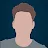ALI Elazkany

immediately uninstalled, the ads are overlapping with the buttons on the app it self, genius!

2.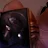demolish everything

Amazing just too many ads but 5 star because I needed this for a test

3.Alka Agarwal

This is a very good app this helps me very much in my studies , I am a school student and this app is excellent

4.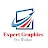Expert Graphics

lot of Thanks this helpfull apps.. i am teaching std 8...9..10 students.. this calc is many help... thanks devloper keep updated apps

5.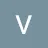Best app I have ever used for calculating Integers

6.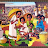ሞገስ

Remove the ads the you get 5*s.

7.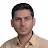nice app, hope to be more user friendly.

8.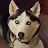static

Better now that ads are gone.

9.Abby Whitefield

Only app that was actually easy to use and had what i needed

10.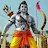Red Leaf

excellent app but not 12 digits